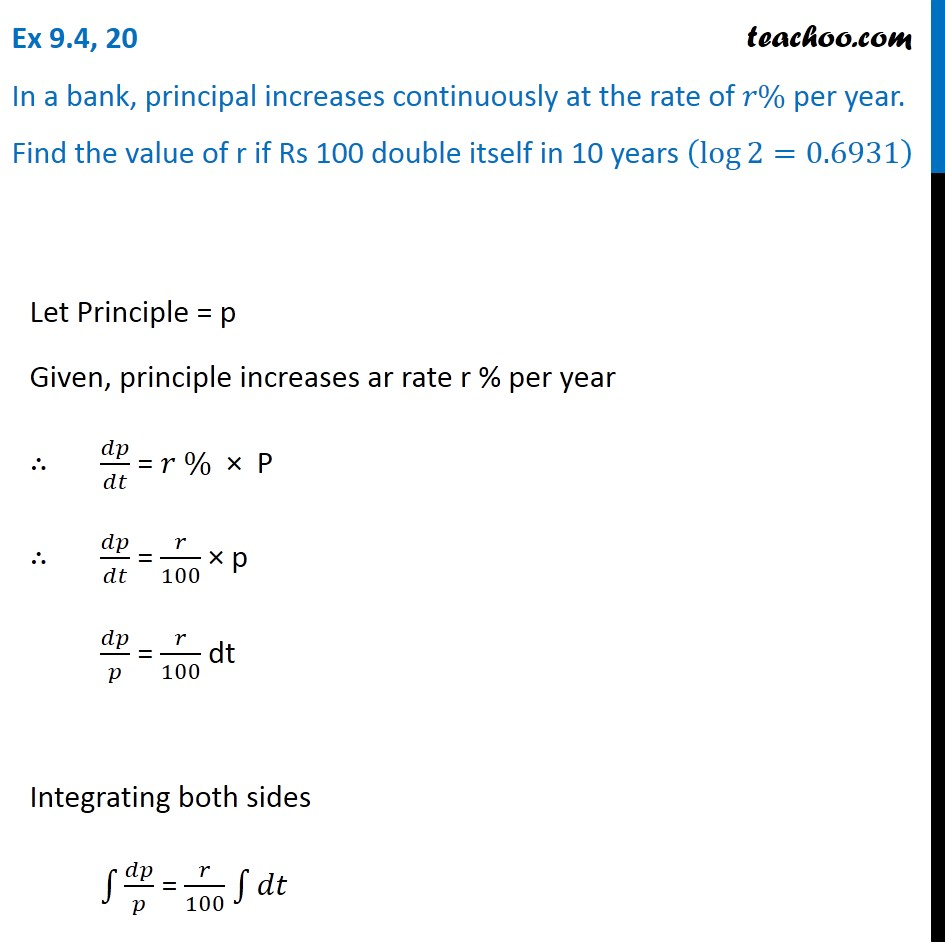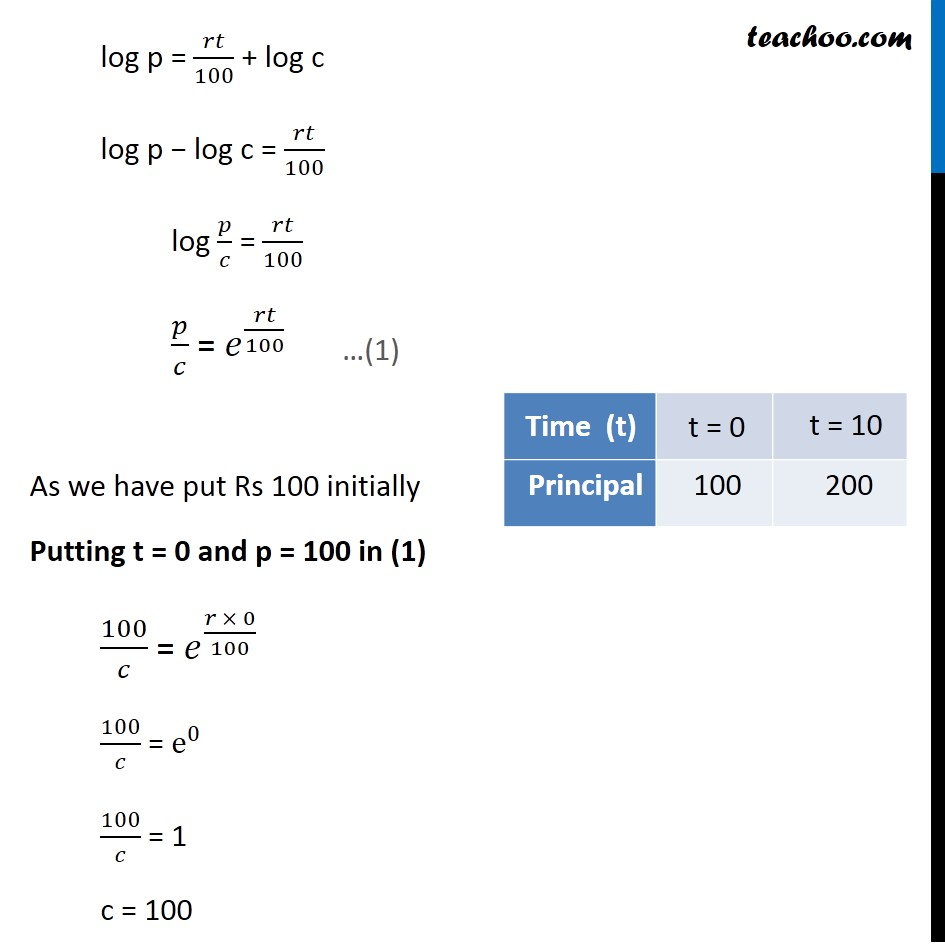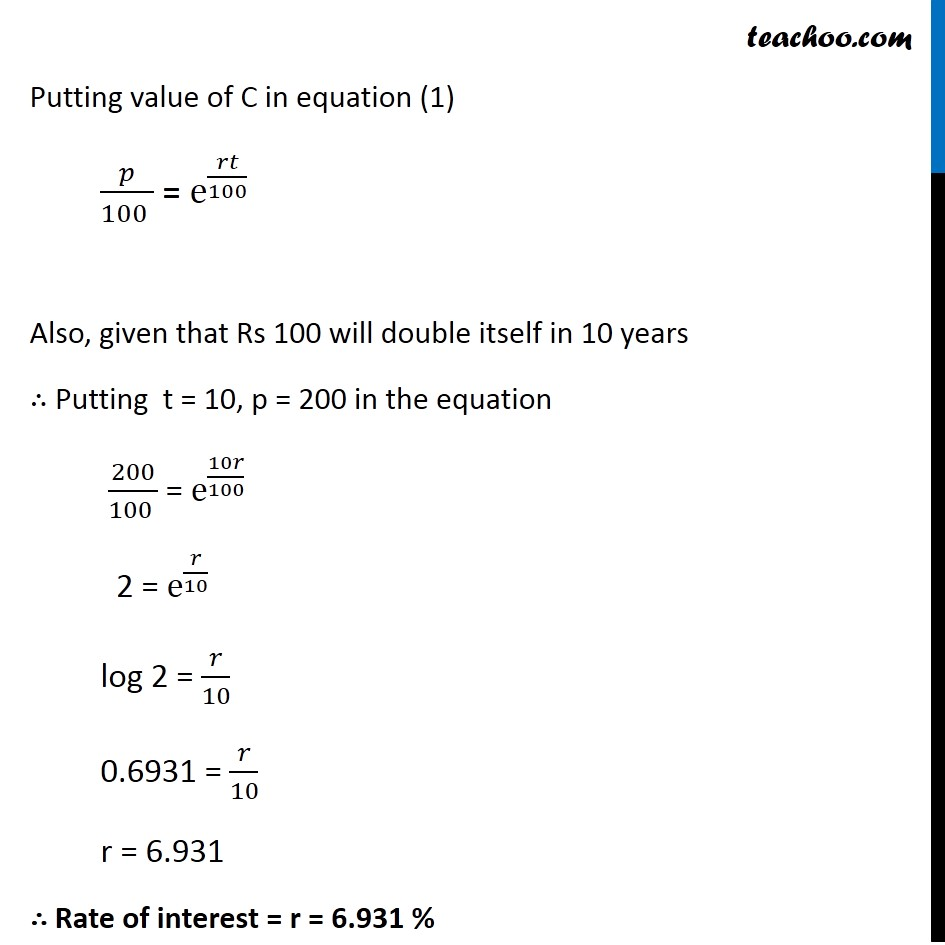Ex 9.3

Chapter 9 Class 12 Differential Equations
Serial order wiseLearn in your speed, with individual attention - Teachoo Maths 1-on-1 Class

### Transcript

Ex 9.3, 20 In a bank, principal increases continuously at the rate of 𝑟% per year. Find the value of r if Rs 100 double itself in 10 years (log⁡〖2=0.6931〗 ) Let Principle = p Given, principle increases ar rate r % per year ∴ 𝑑𝑝/𝑑𝑡 = 𝑟 % × P ∴ 𝑑𝑝/𝑑𝑡 = 𝑟/100 × p 𝑑𝑝/𝑝 = 𝑟/100 dt Integrating both sides ∫1▒𝑑𝑝/𝑝 = 𝑟/100 ∫1▒𝑑𝑡 log p = 𝑟𝑡/100 + log c log p − log c = 𝑟𝑡/100 log 𝑝/𝑐 = 𝑟𝑡/100 𝑝/𝑐 = 𝑒^(𝑟𝑡/100) As we have put Rs 100 initially Putting t = 0 and p = 100 in (1) 100/𝑐 = 𝑒^((𝑟 × 0)/100) 100/𝑐 = e^0 100/𝑐 = 1 c = 100 …(1) Putting value of C in equation (1) 𝑝/(100 ) = e^(𝑟𝑡/100) Also, given that Rs 100 will double itself in 10 years ∴ Putting t = 10, p = 200 in the equation 200/(100 ) = e^(10𝑟/100) 2 = e^(𝑟/10) log 2 = 𝑟/10 0.6931 = 𝑟/10 r = 6.931 ∴ Rate of interest = r = 6.931 %